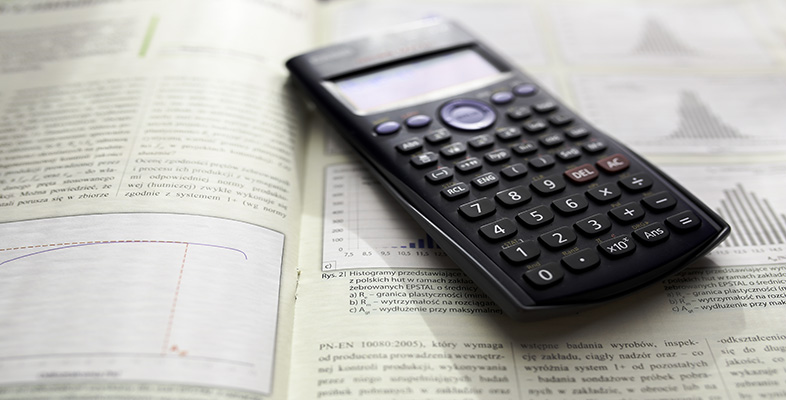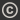Science, Maths & Technology
Free course

# Using a scientific calculator

Free statement of participation on completion## Course reviews

Do you have a Casio fx-83 ES scientific calculator (or a compatible model) and want to learn how to use it? This free course, Using a scientific calculator, will help you to understand how to use the different facilities and functions and discover what a powerful tool this calculator can be!

## Course learning outcomes

After studying this course, you should be able to:

• understand the basic functions on your calculator
• understand which calculator functions are needed for a given problem
• understand what may go wrong when entering calculations and know how to fix them
• apply knowledge of calculator functions to a range of mathematical calculations.

First Published: 10/08/2012

Updated: 10/06/2019

Skip Rate and Review